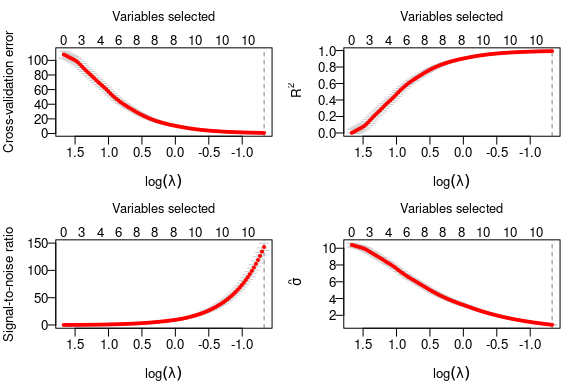1 User Guide

1.1 Small Data

1.1.1 Standar Lasso

library(biglasso)

data(colon)
X <- colon$X y <- colon$y
dim(X)
#>    62 2000
X[1:5, 1:5]
#>   Hsa.3004 Hsa.13491 Hsa.13491.1 Hsa.37254 Hsa.541
#> t  8589.42   5468.24     4263.41   4064.94 1997.89
#> n  9164.25   6719.53     4883.45   3718.16 2015.22
#> t  3825.71   6970.36     5369.97   4705.65 1166.55
#> n  6246.45   7823.53     5955.84   3975.56 2002.61
#> t  3230.33   3694.45     3400.74   3463.59 2181.42
## convert X to a big.matrix object
## X.bm is a pointer to the data matrix
X.bm <- as.big.matrix(X)
str(X.bm)
#> Formal class 'big.matrix' [package "bigmemory"] with 1 slot
dim(X.bm)
#>    62 2000
X.bm[1:5, 1:5]
#>   Hsa.3004 Hsa.13491 Hsa.13491.1 Hsa.37254 Hsa.541
#> t  8589.42   5468.24     4263.41   4064.94 1997.89
#> n  9164.25   6719.53     4883.45   3718.16 2015.22
#> t  3825.71   6970.36     5369.97   4705.65 1166.55
#> n  6246.45   7823.53     5955.84   3975.56 2002.61
#> t  3230.33   3694.45     3400.74   3463.59 2181.42
## same results as X[1:5, 1:5]
## fit entire solution path, using our newly proposed "Adaptive" screening rule (default)
fit <- biglasso(X.bm, y)
plot(fit)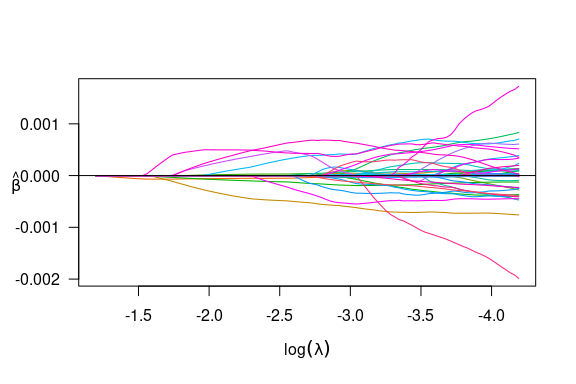1.1.2 Cross-Validation

## 10-fold cross-valiation in parallel
cvfit <- tryCatch(
{
cv.biglasso(X.bm, y, seed = 1234, nfolds = 10, ncores = 4)
},
error = function(cond) {
cv.biglasso(X.bm, y, seed = 1234, nfolds = 10, ncores = 2)
}
)

After cross-validation, a few things we can do:

• plot the cross-validation plots:
par(mfrow = c(2, 2), mar = c(3.5, 3.5, 3, 1) ,mgp = c(2.5, 0.5, 0))
plot(cvfit, type = "all")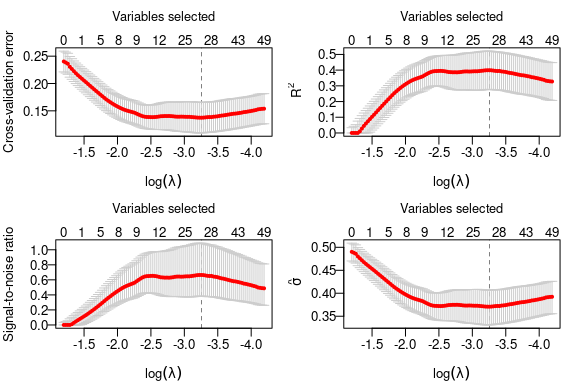• Summarize CV object:
summary(cvfit)
#> lasso-penalized linear regression with n=62, p=2000
#> At minimum cross-validation error (lambda=0.0386):
#> -------------------------------------------------
#>   Nonzero coefficients: 27
#>   Cross-validation error (deviance): 0.14
#>   R-squared: 0.40
#>   Signal-to-noise ratio: 0.66
#>   Scale estimate (sigma): 0.371
• Extract non-zero coefficients at the optimal $$\lambda$$ value:
coef(cvfit)[which(coef(cvfit) != 0),]
#>   (Intercept)      Hsa.8147     Hsa.43279     Hsa.36689      Hsa.3152
#>  6.882526e-01 -5.704059e-07 -2.748858e-08 -6.967419e-04  4.940698e-05
#>     Hsa.36665     Hsa.692.2      Hsa.1272       Hsa.166     Hsa.31801
#>  1.297733e-05 -1.878545e-04 -1.808689e-04  3.717512e-04  1.119437e-04
#>      Hsa.3648      Hsa.1047     Hsa.13628      Hsa.3016      Hsa.5392
#>  1.508691e-04  6.557284e-07  6.519466e-05  2.479566e-05  5.741251e-04
#>      Hsa.1832      Hsa.1464     Hsa.12241     Hsa.44244      Hsa.9246
#> -4.052627e-05  1.821951e-05 -1.912212e-04 -3.369856e-04 -1.582765e-06
#>     Hsa.41159     Hsa.33268      Hsa.6814      Hsa.1660       Hsa.404
#>  3.974870e-04 -4.911208e-04  5.639023e-04  5.171245e-04 -5.208537e-05
#>     Hsa.43331      Hsa.1491   Hsa.41098.1
#> -6.853944e-04  2.977285e-04 -1.748628e-04

1.1.3 Logistic Regression

data(Heart)
X <- Heart$X y <- Heart$y
X.bm <- as.big.matrix(X)
fit <- biglasso(X.bm, y, family = "binomial")
plot(fit)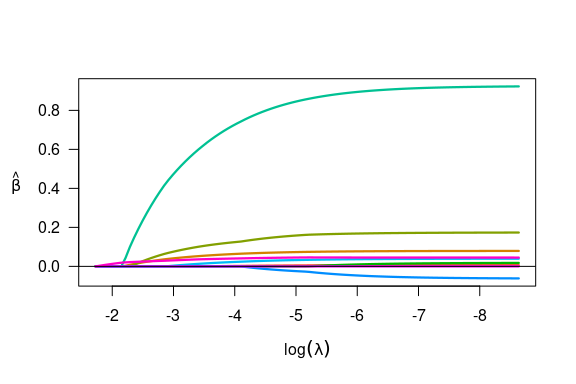1.1.4 Cox Regression

library(survival)
#>
#> Attaching package: 'survival'
#> The following object is masked _by_ '.GlobalEnv':
#>
#>     colon
#> The following object is masked from 'package:ncvreg':
#>
#>     heart
X <- heart[,4:7]
y <- Surv(heart$stop - heart$start, heart\$event)
X.bm <- as.big.matrix(X)
#> Warning in as.big.matrix(X): Coercing data.frame to matrix via factor level
#> numberings.
fit <- biglasso(X.bm, y, family = "cox")
plot(fit)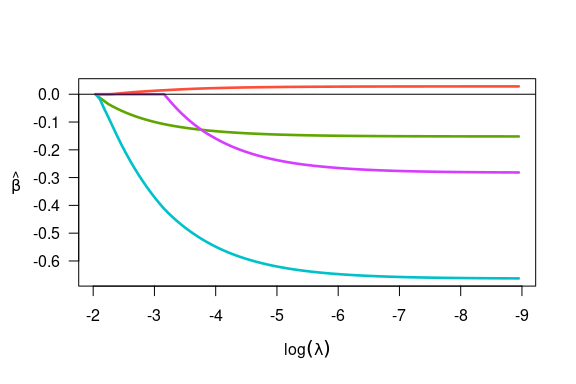1.2 Big Data

When the raw data file is very large, it’s better to convert the raw data file into a file-backed big.matrix by using a file cache. We can call function setupX, which reads the raw data file and creates a backing file (.bin) and a descriptor file (.desc) for the raw data matrix:

## The data has 1000 observations and 5,000 features.
## Much larger data can be handled in the same way.
## 10 of the features has non-zero coefficients
if(!file.exists('BigX.bin')) {
X <- matrix(rnorm(1000 * 5000), 1000, 5000)
beta <- c(-5:5)
y <- as.numeric(X[,1:11] %*% beta)
write.csv(X, "BigX.csv", row.names = F)
write.csv(y, "y.csv", row.names = F)
## Pretend the data is stored in the ~90MB .csv file and is too large to fit into memory
X.bm <- setupX("BigX.csv", header = T)
}
#> Reading data from file, and creating file-backed big.matrix...
#> This should take a while if the data is very large...
#> Start time:  2021-01-30 16:06:38
#> End time:  2021-01-30 16:06:41
#> DONE!
#>
#> Note: This function needs to be called only one time to create two backing
#>       files (.bin, .desc) in current dir. Once done, the data can be
#>       'loaded' using function 'attach.big.matrix'. See details in doc.

It’s important to note that the above operation is just one-time execution. Once done, the data can always be retrieved seamlessly by attaching its descriptor file (.desc) in any new R session:

rm(list = c("X", "X.bm", "y")) # Pretend starting a new session
X.bm <- attach.big.matrix("BigX.desc")

This is very appealing for big data analysis in that we don’t need to “read” the raw data again in a R session, which would be very time-consuming. The code below again fits a lasso-penalized linear model, and runs 10-fold cross-validation:

system.time({fit <- biglasso(X.bm, y)})
#>    user  system elapsed
#>   0.211   0.002   0.213
plot(fit)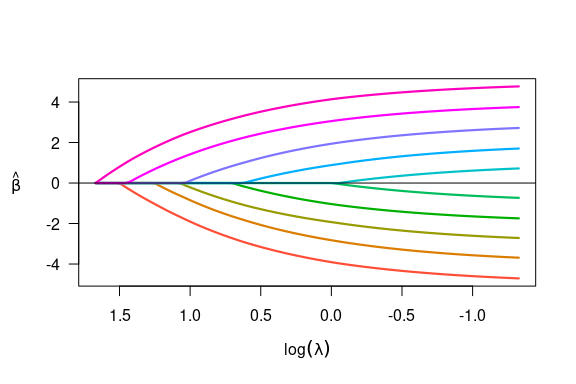# 10-fold cross validation in parallel
tryCatch(
{
system.time({cvfit <- cv.biglasso(X.bm, y, seed = 1234, ncores = 4, nfolds = 10)})
},
error = function(cond) {
system.time({cvfit <- cv.biglasso(X.bm, y, seed = 1234, ncores = 2, nfolds = 10)})
}
)
#>    user  system elapsed
#>   0.337   0.005   3.011
par(mfrow = c(2, 2), mar = c(3.5, 3.5, 3, 1), mgp = c(2.5, 0.5, 0))
plot(cvfit, type = "all")